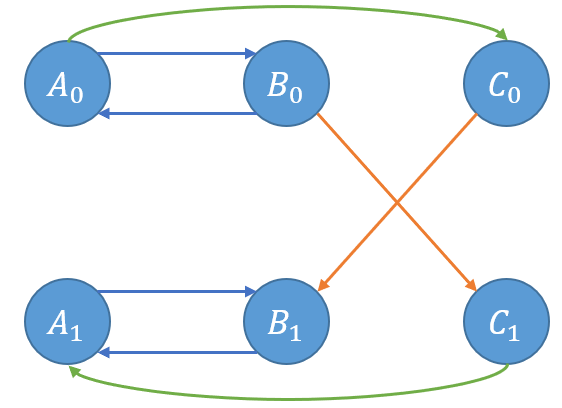# 2-SAT问题【学习笔记】

2-SAT问题是用于解决当每个元素有两个状态（0或1），且有约束关系时的问题。

首先分析一下问题模型：给定3个元素，需要从中选出几个，其中

1. $A,B$同时被选或同时不选；
2. $B$和$C$必须被选一个；
3. $A$被选或$C$不选。

我们可以把问题的模型建立出来，因为它们有约束关系，也就是一个元素的状态会影响到另一个元素。我们设选择这个元素的状态是1，不选为0。做这种题，如果建立并查集模型，我们可以把一个点拆成0,1两部分。我们知道这里的条件是单方面的，也就是充分条件，单独考虑一条有向边，则箭头的终点无法决定箭头的起点，这符合2-SAT的特性，因此可以用有向图来建模。

在上面的模型中，我们可以建出这样一个图来：其中蓝色边代表限制1，橙色边代表限制2，绿色边代表限制3。比如对于橙色边，表示当B没有被选时，C就要被选，当C没有被选时，B就要被选。也就是满足了限制2。

在这样的有向图中，是很容易成环的。容易发现，当某个点的0,1两部分同在一个强连通分量中，就说明它们冲突了，表示互相约束。此时题目无解。其他情况时，我们应该先选拓扑序大的，也就是后进队的，因为这样的节点不会去限制后面的节点。当一个位置的两个状态存在单向边关系（不一定是直接边）时，我们就要这样选。

事实上我们不需要再进行一边拓扑排序，只需要选择两个状态中强连通分量编号小的那一个就可以了，编号顺序就是强连通分量被找到的顺序。当两个节点在同一条链上时，会互相约束，因此选编号小的；如果不在同一条链上，那么选哪一个都可以。因此我们就解决了2-SAT问题并任意输出了方案。

如果要求字典序最小，那么就要尽量使得较小的点为0，然后枚举时把链上的点全部更新一遍就可以了但是我还没写咕咕咕

## Code：

#include<cstdio>
#include<cstring>
struct edge
{
int n,nxt;
edge(int n,int nxt)
{
this->n=n;
this->nxt=nxt;
}
edge(){}
}e;
{
}
int dfn,low,cnt=0;
bool in;
int stk,tp=0,del,dcnt=0;
void tarjan(int x)
{
dfn[x]=++cnt;
low[x]=dfn[x];
in[x]=1;
stk[++tp]=x;
if(dfn[e[i].n])
{
if(in[e[i].n])
low[x]=low[x]<dfn[e[i].n]?low[x]:dfn[e[i].n];
}
else
{
tarjan(e[i].n);
low[x]=low[x]<low[e[i].n]?low[x]:low[e[i].n];
}
if(dfn[x]==low[x])
{
++dcnt;//依次编号
do
{
del[stk[tp]]=dcnt;
in[stk[tp--]]=0;
}while(stk[tp+1]!=x);
}
return;
}
int ans,n;
int main()
{
memset(ans,-1,sizeof(ans));
int m,u,v,w,x;
scanf("%d%d",&n,&m);
for(int i=1;i<=m;++i)
{
scanf("%d%d%d%d",&u,&v,&w,&x);//0+u表示不选 n+u表示选
}
for(int i=1;i<=2*n;++i)
if(!dfn[i])
tarjan(i);
for(int i=1;i<=n;++i)
if(del[i]==del[i+n])
{
puts("IMPOSSIBLE");
return 0;
}
puts("POSSIBLE");
for(int i=1;i<=n;++i)
printf("%d ",del[i]<del[i+n]?0:1);//选择强连通分量编号较小的
return 0;
}

### 2 说点什么0 Followers

Most reacted comment
0 Comment authors
Recent comment authors
Subscribe[…] 【2-SAT学习笔记】 […]wpDiscuz